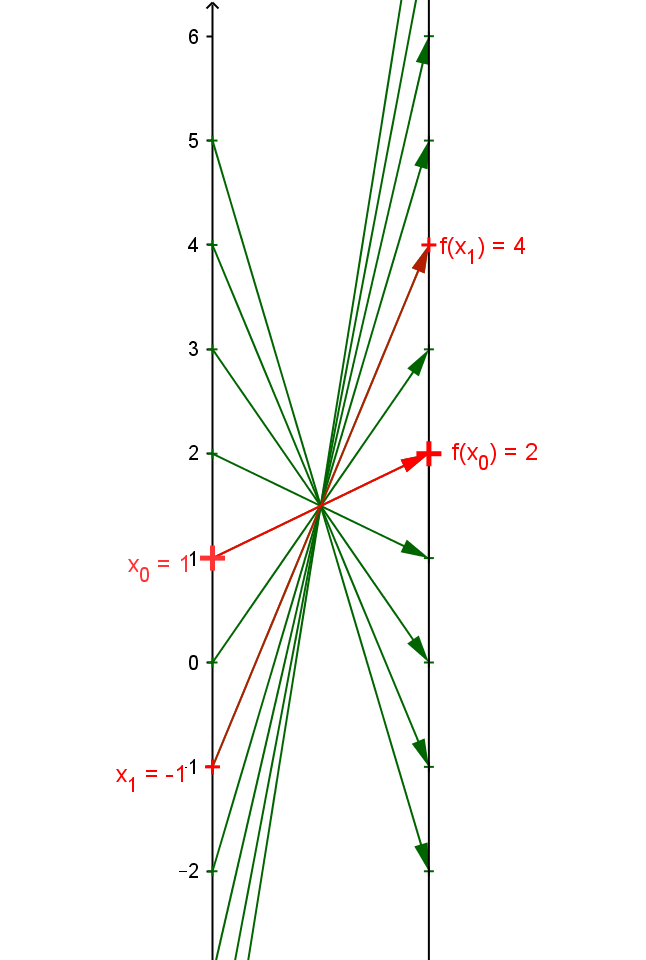Example LF.FORM.3 : Suppose $f$ is a linear function with $f(1) = 2$ and $f(-1) = 4$. Find the slope and then  point-slope form of the line.
Visualize $f$ with a mapping diagram that illustrates the slope = $m$ with $f(-1) = 2$ and $f(1) =4$.
Find the X- and Y-intercepts for $f$ and visualize them on the mapping diagram as well.
Explain how to find the focus point for $f$ and how this can be used to find the intercepts and slope without using any of the forms or equation to find slope.

Solution: The slope is the rate and magnification factor. $m = \frac {\Delta y} {\Delta x} = \frac {f(1)-f(-1)} {1 - (-1)} = \frac {2-4} 2 = -1$ .
We can use the point slope form with $m=-1$ and $f(1)=2$ to obtain $f(x) = 2 - 1*(x-1) = - x + 3.$
This is confirmed by checking $f(-1) = 2 - (-1-1) = 4$.
You can now work the remainder of the algebra part of problem as with the other examples.

Draw a mapping diagram yourself or use the GeoGebra figure.Given a point / number, $x$, on the source line, there is a unique arrow  meeting the target line at the point / number, $2 - 1*(x-1) = -x + 3$, which corresponds to the linear function's value for $x$

When the point in the domain is $-1$, the arrow points to $f(-1) = 4$ visualizing the point $(-1,4)$ on the graph of $f$.
We also have $f(1)= 2$ so the difference in the value of $f$ for -2 units change in $x$ is $\Delta y = f(1)-f(-1) = 4-2=2.$
Since a unit step is used in this mapping diagram, we see the slope (magnification, rate) visualized in the gap between the heads of consecutive arrows on the mapping diagram.
As  $m < 0$, the arrows cross at a focus point between the two axes, consistent with $=m=-1$ and that the function is decreasing  at a rate of one unit on the target value for every unit increase in the domain value.

An arrow through $x=0$ and the focus point finds $f(0) = 3$.
The X-intercept is the value $a$ on the domain axis from which the arrow hits the number $0$  on the target.
For this function, that value is $a= 3$. You can check this by entering $3$ for $a$ on the GeoGebra figure.
An arrow through the focus point with its head at $0$ on the target comes from $x=3$ on the domain axis.